## Introduction to mechanics¶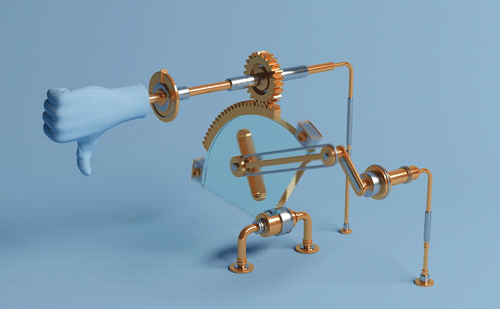### Concept of degrees of freedom (DOF)¶

In physics, the degree of freedom (DOF) of a mechanical system is the number of independent parameters that define its configuration. It is the number of parameters that determine the state of a physical system and is important to the analysis of systems of bodies in mechanical engineering, aeronautical engineering, robotics, and structural engineering. The degrees of freedom can be calculated depending on the type of joints and support that a mechanism has. As a summary:

• If DOF = 1 -> Deterministic mechanism
• If DOF = 0 -> Structure
• If DOF > 1 -> Non-deterministic mechanismThings can get more difficult to analyse:And structures can get complex:### Mechanisms¶

• Linear movement:

• Linear bearings / guides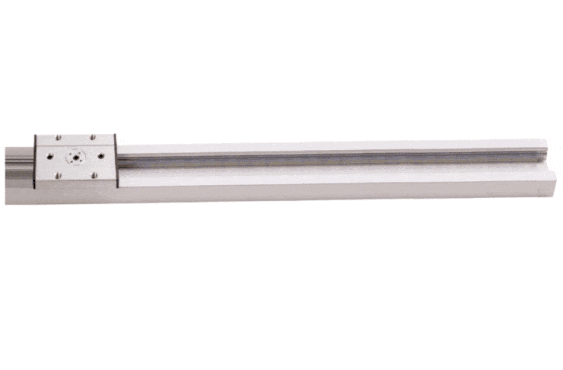• Frictive mechanisms (piston)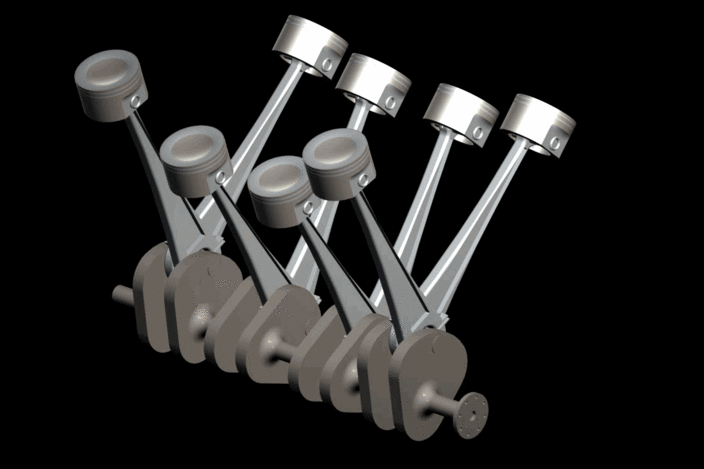• Screw• Rotational movement:

When things need to have a coupled rotation, we can use gears, belts or chains to transfer the movement to one another. In any of them, we define transfer ratio as the ratio between the rotational speeds. If we consider the number of teeth of a gear, or pinion as r, and its speed as w:

i = w2/w1 = r1/r2

#### Gears¶

When things are close to each other: we use gears:• Straight gear: simple, but noisy• Helix gear: more complex, but silent:• Straight Bevel gear: when we have angles• Helix Bevel gear: all of the above• Pinion-rack: from linear to rotation• Planetary gears!#### Belts and chains¶

When distances are longer, we don’t use gears due to it’s size, we use either belts or chains. Chains can transmit more power, but they are more noisy and they don’t absorbe as much sudden vibrations as belts:

• Chain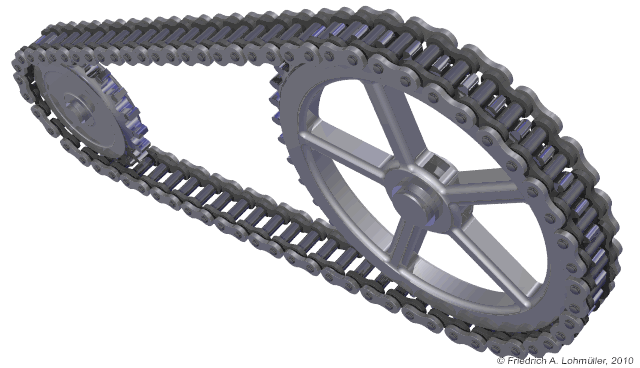• BeltAn continuosly variable transmission (CVT):#### Cams¶

Cams can come in different flavours: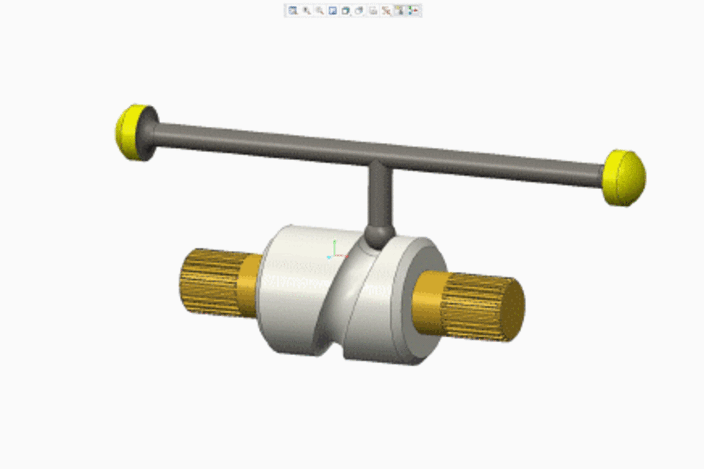### Keeping things in place¶

• Bearings

• Linear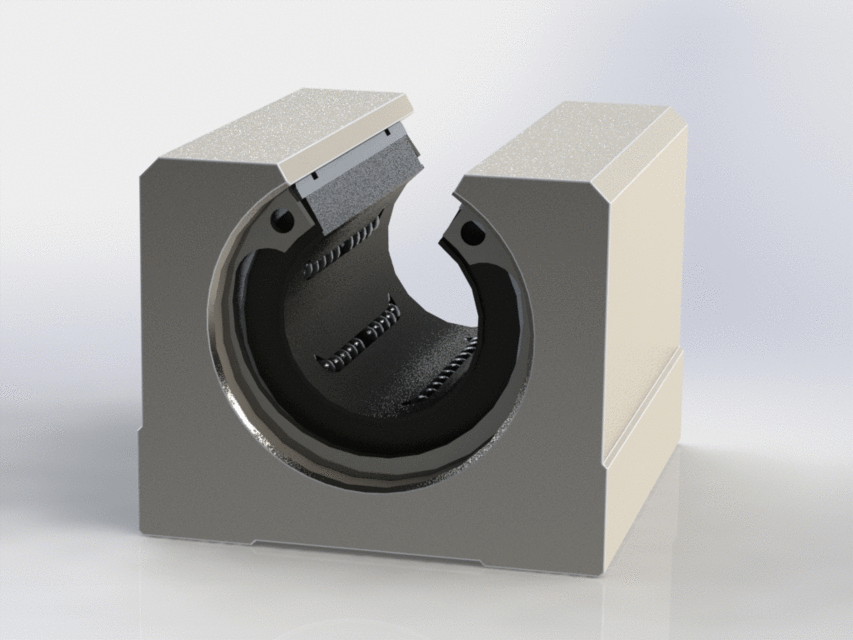• Ball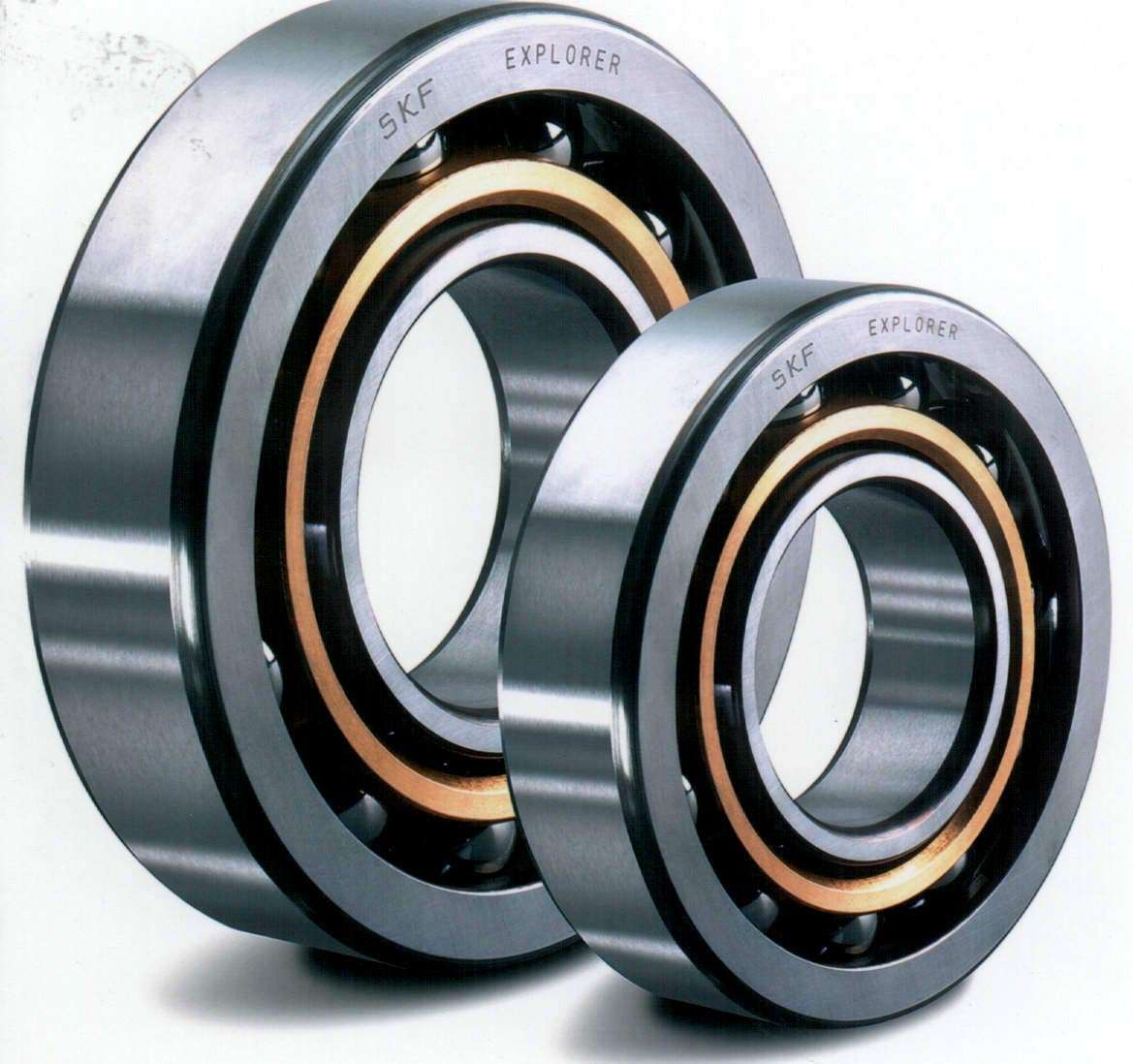• Flat/needle• Conical• Rings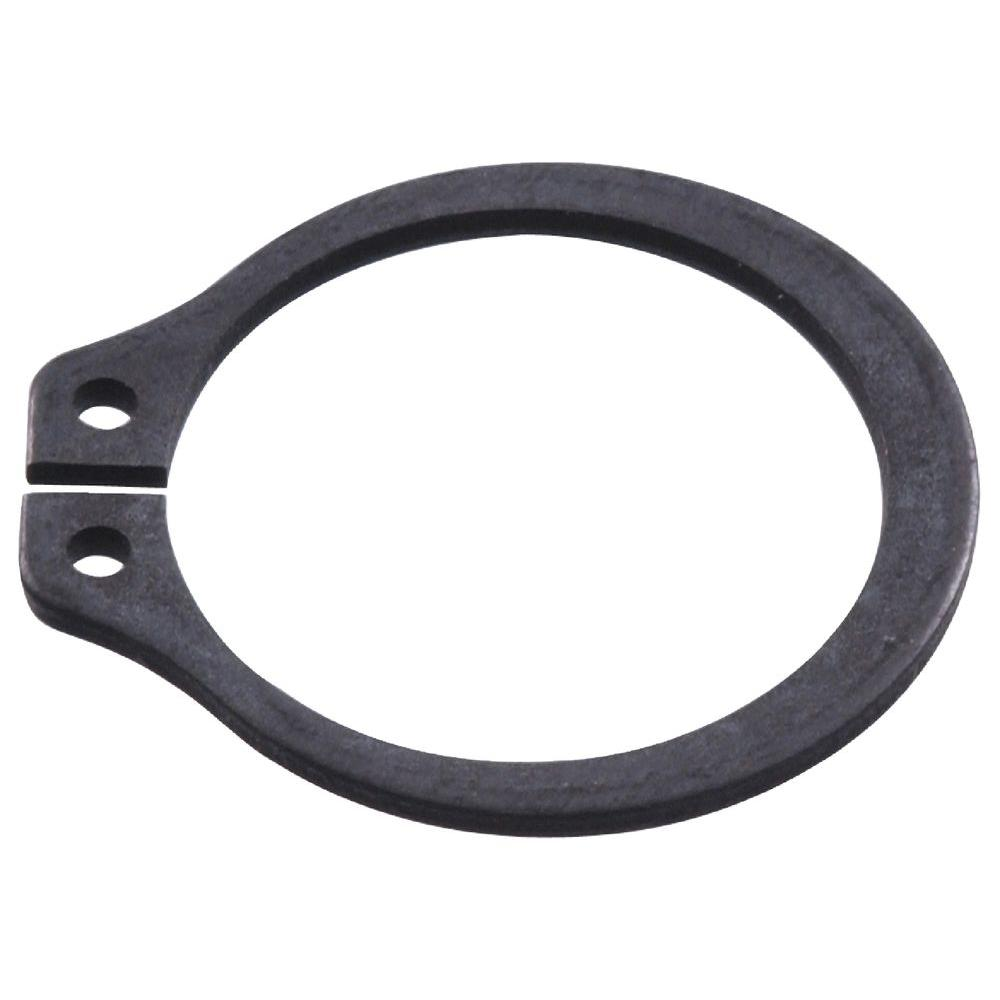### Mechanisms examples¶

• Bars mechanisms:

• 4-bar mechanism: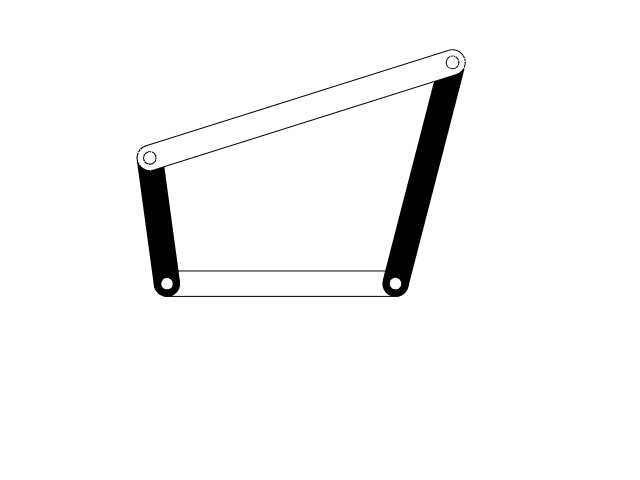``- Locking plyers````- Crane````- Excavator``• Ratchet• Geneva wheel• Universal joint• Core X/Y